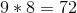# ISEE Middle Level Math : How to find the square root

## Example Questions

### Example Question #71 : Squares / Square Roots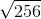Explanation:

Find the square root: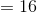### Example Question #72 : Squares / Square Roots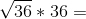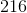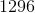Explanation:

First, find the square root: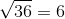Then, solve: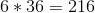### Example Question #73 : Squares / Square Roots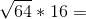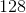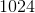Explanation:

First, find the square root: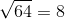Then, solve: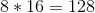### Example Question #74 : Squares / Square Roots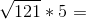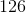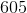Explanation:

First, find the square root: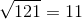Then, solve: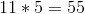### Example Question #75 : Squares / Square Roots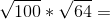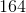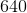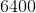Explanation:

First, find the square roots: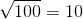Then, solve: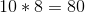### Example Question #76 : Squares / Square Roots

Evaluate: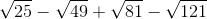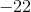Explanation: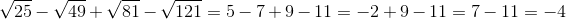### Example Question #77 : Squares / Square Roots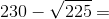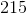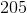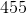Explanation:

First, find the square root: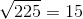Then solve: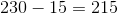### Example Question #78 : Squares / Square Roots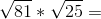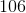Explanation:

First, find the square roots: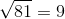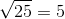Then, solve: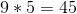### Example Question #79 : Squares / Square Roots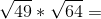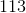Explanation:

First, find the square roots: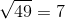Then, solve: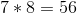### Example Question #80 : Squares / Square Roots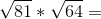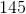Explanation:

First, find the square roots:Then, solve: